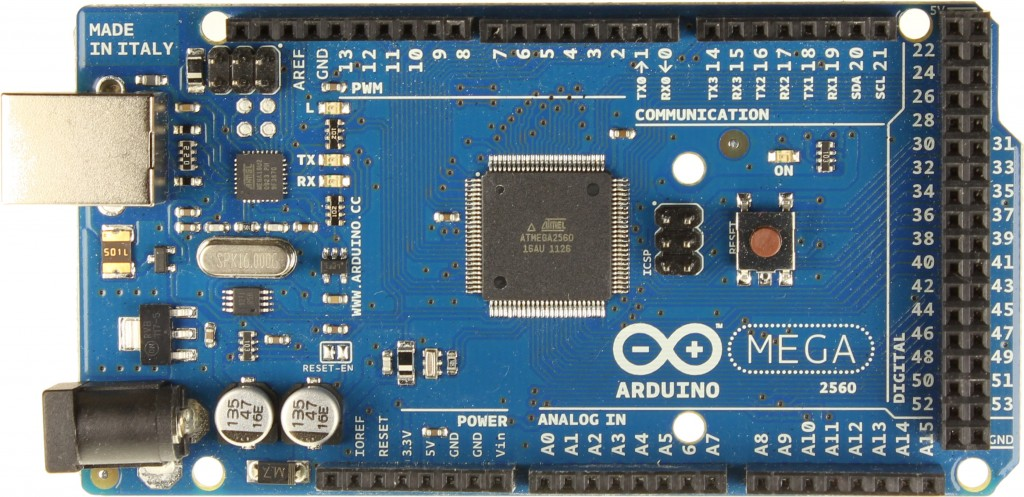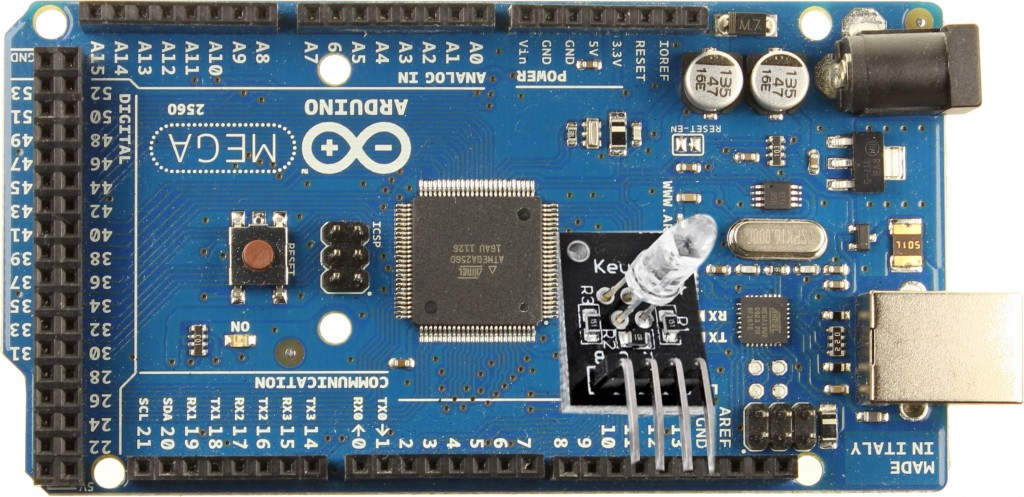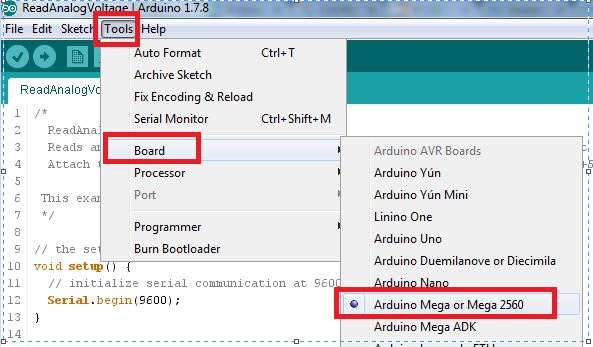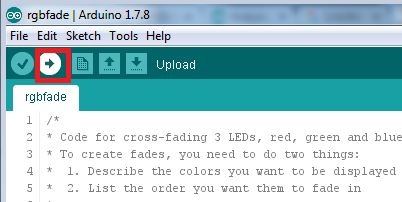# RGB LED Fading Using Arduino Board and KEYES RGB LED

By | February 5, 2016

Parts Required :

• Arduino Board• Keyes RGB LED Module (http://www.dx.com/p/diy-arduino-3-color-rgb-smd-led-module-black-135046#.VrTWlvl96M8)Connect the RGB to Pin 11,12,13,GND as below.If you connect like above you no need to use any cables. Sinces the RGB mini board already contains built in resistors , you can use this kit out of box.

https://www.arduino.cc/en/Main/Software

Once you download , connect Arduino board to PC and open the Arduino IDE

Now find out the port used by the Arduino from the device manager of PC. Below image shows that my board is using COM 3Open Arduino IDE , go to Tools –> Port — Select COM3 as shown below.Now from Tools –> Board –> Arduino Mega

If you are using other board , select your board model.Now Paste the below code to your IDE ..

```/*
* Code for cross-fading 3 LEDs, red, green and blue (RGB)
* To create fades, you need to do two things:
* 1. Describe the colors you want to be displayed
* 2. List the order you want them to fade in
*
* DESCRIBING A COLOR:
* A color is just an array of three percentages, 0-100,
* controlling the red, green and blue LEDs
*
* Red is the red LED at full, blue and green off
* int red = { 100, 0, 0 }
* Dim white is all three LEDs at 30%
* int dimWhite = {30, 30, 30}
* etc.
*
* Some common colors are provided below, or make your own
*
* LISTING THE ORDER:
* In the main part of the program, you need to list the order
* you want to colors to appear in, e.g.
*
* Those colors will appear in that order, fading out of
* one color and into the next
*
* 1. The initial color is set to black (so the first color fades in), but
* you can set the initial color to be any other color
* 2. The internal loop runs for 1020 interations; the 'wait' variable
* sets the approximate duration of a single crossfade. In theory,
* a 'wait' of 10 ms should make a crossFade of ~10 seconds. In
* practice, the other functions the code is performing slow this
* down to ~11 seconds on my board. YMMV.
* 3. If 'repeat' is set to 0, the program will loop indefinitely.
* if it is set to a number, it will loop that number of times,
* then stop on the last color in the sequence. (Set 'return' to 1,
* and make the last color black if you want it to fade out at the end.)
* 4. There is an optional 'hold' variable, which pasues the
* program for 'hold' milliseconds when a color is complete,
* but before the next color starts.
* 5. Set the DEBUG flag to 1 if you want debugging output to be
* sent to the serial monitor.
*
* The internals of the program aren't complicated, but they
* are a little fussy -- the inner workings are explained
* below the main loop.
*
* April 2007, Clay Shirky <[email protected]>
*/

// Output
int redPin = 12; // Red LED, connected to digital pin 9
int grnPin = 13; // Green LED, connected to digital pin 10
int bluPin = 11; // Blue LED, connected to digital pin 11

// Color arrays
int black = { 0, 0, 0 };
int white = { 100, 100, 100 };
int red = { 100, 0, 0 };
int green = { 0, 100, 0 };
int blue = { 0, 0, 100 };
int yellow = { 40, 95, 0 };
int dimWhite = { 30, 30, 30 };
// etc.

// Set initial color
int redVal = black;
int grnVal = black;
int bluVal = black;

int wait = 10; // 10ms internal crossFade delay; increase for slower fades
int hold = 0; // Optional hold when a color is complete, before the next crossFade
int DEBUG = 1; // DEBUG counter; if set to 1, will write values back via serial
int loopCount = 60; // How often should DEBUG report?
int repeat = 3; // How many times should we loop before stopping? (0 for no stop)
int j = 0; // Loop counter for repeat

// Initialize color variables
int prevR = redVal;
int prevG = grnVal;
int prevB = bluVal;

// Set up the LED outputs
void setup()
{
pinMode(redPin, OUTPUT); // sets the pins as output
pinMode(grnPin, OUTPUT);
pinMode(bluPin, OUTPUT);

if (DEBUG) { // If we want to see values for debugging...
Serial.begin(9600); // ...set up the serial ouput
}
}

// Main program: list the order of crossfades
void loop()
{

if (repeat) { // Do we loop a finite number of times?
j += 1;
if (j >= repeat) { // Are we there yet?
exit(j); // If so, stop.
}
}
}

/* BELOW THIS LINE IS THE MATH -- YOU SHOULDN'T NEED TO CHANGE THIS FOR THE BASICS
*
* The program works like this:
* Imagine a crossfade that moves the red LED from 0-10,
* the green from 0-5, and the blue from 10 to 7, in
* ten steps.
* We'd want to count the 10 steps and increase or
* decrease color values in evenly stepped increments.
* Imagine a + indicates raising a value by 1, and a -
* equals lowering it. Our 10 step fade would look like:
*
* 1 2 3 4 5 6 7 8 9 10
* R + + + + + + + + + +
* G + + + + +
* B - - -
*
* The red rises from 0 to 10 in ten steps, the green from
* 0-5 in 5 steps, and the blue falls from 10 to 7 in three steps.
*
* In the real program, the color percentages are converted to
* 0-255 values, and there are 1020 steps (255*4).
*
* To figure out how big a step there should be between one up- or
* down-tick of one of the LED values, we call calculateStep(),
* which calculates the absolute gap between the start and end values,
* and then divides that gap by 1020 to determine the size of the step
* between adjustments in the value.
*/

int calculateStep(int prevValue, int endValue) {
int step = endValue - prevValue; // What's the overall gap?
if (step) { // If its non-zero,
step = 1020/step; // divide by 1020
}
return step;
}

/* The next function is calculateVal. When the loop value, i,
* reaches the step size appropriate for one of the
* colors, it increases or decreases the value of that color by 1.
* (R, G, and B are each calculated separately.)
*/

int calculateVal(int step, int val, int i) {

if ((step) && i % step == 0) { // If step is non-zero and its time to change a value,
if (step > 0) { // increment the value if step is positive...
val += 1;
}
else if (step < 0) { // ...or decrement it if step is negative
val -= 1;
}
}
// Defensive driving: make sure val stays in the range 0-255
if (val > 255) {
val = 255;
}
else if (val < 0) {
val = 0;
}
return val;
}

/* crossFade() converts the percentage colors to a
* 0-255 range, then loops 1020 times, checking to see if
* the value needs to be updated each time, then writing
* the color values to the correct pins.
*/

// Convert to 0-255
int R = (color * 255) / 100;
int G = (color * 255) / 100;
int B = (color * 255) / 100;

int stepR = calculateStep(prevR, R);
int stepG = calculateStep(prevG, G);
int stepB = calculateStep(prevB, B);

for (int i = 0; i <= 1020; i++) {
redVal = calculateVal(stepR, redVal, i);
grnVal = calculateVal(stepG, grnVal, i);
bluVal = calculateVal(stepB, bluVal, i);

analogWrite(redPin, redVal); // Write current values to LED pins
analogWrite(grnPin, grnVal);
analogWrite(bluPin, bluVal);

delay(wait); // Pause for 'wait' milliseconds before resuming the loop

if (DEBUG) { // If we want serial output, print it at the
if (i == 0 or i % loopCount == 0) { // beginning, and every loopCount times
Serial.print("Loop/RGB: #");
Serial.print(i);
Serial.print(" | ");
Serial.print(redVal);
Serial.print(" / ");
Serial.print(grnVal);
Serial.print(" / ");
Serial.println(bluVal);
}
DEBUG += 1;
}
}
// Update current values for next loop
prevR = redVal;
prevG = grnVal;
prevB = bluVal;
delay(hold); // Pause for optional 'wait' milliseconds before resuming the loop
}```

Now Click Upload as shown below to upload the code to the board.Now your LED start fading with various colours. You can also change the color settings as per comments in the code.